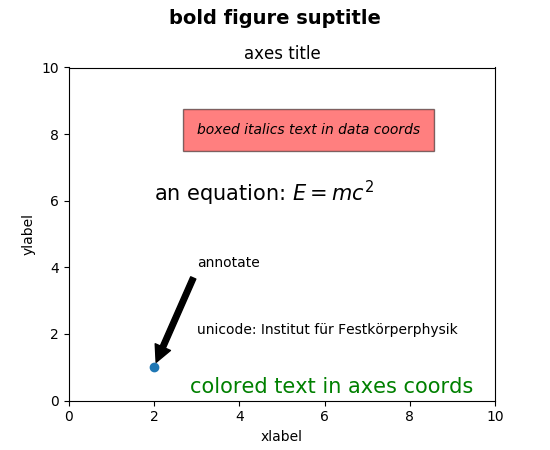Travis-CI:# Text introduction¶

matplotlib has extensive text support, including support for mathematical expressions, truetype support for raster and vector outputs, newline separated text with arbitrary rotations, and unicode support. Because it embeds fonts directly in output documents, e.g., for postscript or PDF, what you see on the screen is what you get in the hardcopy. FreeType support produces very nice, antialiased fonts, that look good even at small raster sizes. matplotlib includes its own `matplotlib.font_manager` (thanks to Paul Barrett), which implements a cross platform, `W3C` compliant font finding algorithm.

The user has a great deal of control over text properties (font size, font weight, text location and color, etc.) with sensible defaults set in the `rc file`. And significantly, for those interested in mathematical or scientific figures, matplotlib implements a large number of TeX math symbols and commands, supporting mathematical expressions anywhere in your figure.

# Basic text commands¶

The following commands are used to create text in the pyplot interface

All of these functions create and return a `matplotlib.text.Text()` instance, which can be configured with a variety of font and other properties. The example below shows all of these commands in action.

```# -*- coding: utf-8 -*-
import matplotlib.pyplot as plt

fig = plt.figure()
fig.suptitle('bold figure suptitle', fontsize=14, fontweight='bold')

ax.set_title('axes title')

ax.set_xlabel('xlabel')
ax.set_ylabel('ylabel')

ax.text(3, 8, 'boxed italics text in data coords', style='italic',

ax.text(2, 6, r'an equation: \$E=mc^2\$', fontsize=15)

ax.text(3, 2, u'unicode: Institut f\374r Festk\366rperphysik')

ax.text(0.95, 0.01, 'colored text in axes coords',
verticalalignment='bottom', horizontalalignment='right',
transform=ax.transAxes,
color='green', fontsize=15)

ax.plot(, , 'o')
ax.annotate('annotate', xy=(2, 1), xytext=(3, 4),
arrowprops=dict(facecolor='black', shrink=0.05))

ax.axis([0, 10, 0, 10])

plt.show()
```